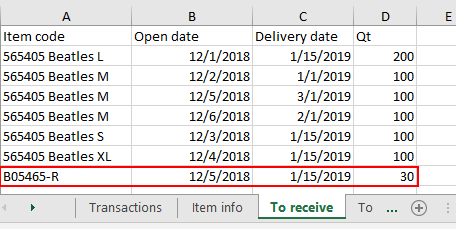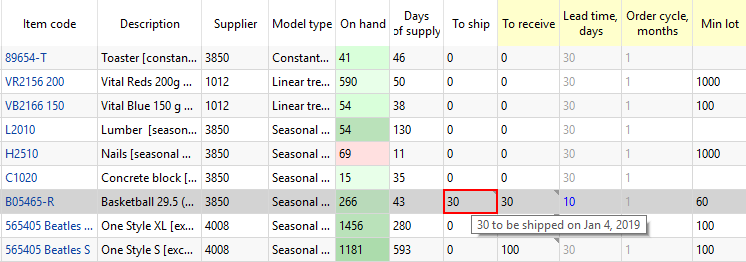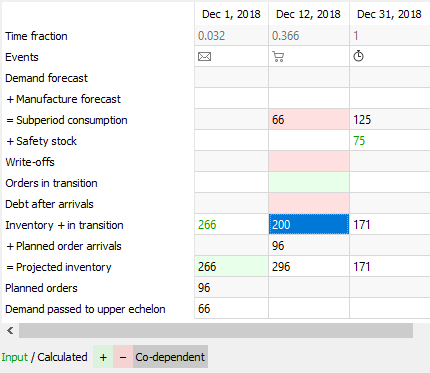#User Guide | GMDH Streamline

### Site Tools

Action disabled: source
ordering-plan-calculation-general

# 6.4.1. Inventory Planning in General

The formulas below refer to calculation an ordering plan for planning items that are not distributed in DCs. In other words, demand for these planning items is generated by selling activity, not distribution. Ordering plan calculation for planning items that are distributed is described in the Two-echelon planning article.

To calculate the first planned order, Streamline uses the following Excel-like formula:

Order qty1 = MAX(CEILING(MAX(0, DOC + Safety stock + Qty_to_shipLT,OC - Remaining), Rounding), Min lot) (1),

Remaining = MAX(0, MAX(0, On hand) + Qty_to_receiveLT,OC - DLT),

where:

• DOC – the demand forecast during the Order cycle coming after the Lead time.
• DLT – the demand forecast for the Lead time period.
• Qty_to_shipLT,OC and Qty_to_receiveLT,OC – the quantities that are to be shipped and received correspondingly within the Lead time plus Order cycle period. Those can be viewed in the In transition details dialog.
• On hand, Order cycle, Lead time, Rounding, Min lot – replenishment parameters shown in the Inventory planning tab.

The calculated Order qty1 is shown in the Qty column of the Current order section on the Inventory planning tab.

To calculate replenishment orders for the next order cycles, the following Excel-like formula is used:

Order qtyi = MAX(CEILING(MAX(0, D(OCi) + Safety stocki + Qty_to_ship(OCi) - Qty_to_receive(OCi) - Remainingi-1), Rounding), Min lot), `i = 2,…`, (2)

where:

• D(OCi) – the demand during `i`-th Order cycle period.
• Safety stocki – the calculated safety stock for `i`-th Order cycle period.
• Qty_to_ship(OCi) and Qty_to_receive(OCi) – the amounts of inventory that are to be shipped and received correspondingly within `i`-th Order cycle period.
• Remainingi-1 – the remaining on-hand at the end of the previous Order cycle period.

To demonstrate how Streamline calculates an ordering plan, we will use the built-in example Inventory Planning by Month. We have slightly changed the input data of the project:

• added a customer order that is to be shipped on Jan 4 of 2019;
• added a line into the To receive worksheet of the Excel document used as the data source for this example (see figure below);
• changed the default Lead time to 10 days for item B05465-R (see figure below); and
• set Streamline to take safety stock as demand for the future month.## Ordering plan

As we mentioned above, an ordering plan is calculated in two steps. First, the current order quantity is computed.

To figure out how the current order of 96 units is obtained, we will address the Explain inventory calculation dialog. To open it, we set the cursor at item B05465-R and press Ctrl + E (see figure below).

As Lead time = 10 days that ends up on Dec 12, 2018 and the Order cycle is 1 month, we take the Subperiod consumption demand starting from the end of Dec 12, 2018 to the end of Jan 12, 2019. Since the dialog shows the dates the particular subperiod ends, we take the sum for the four subperiods staring from Dec 31, 2018.

• Thus, DOC = 125 + 3 + 10 + 24 = 162.
• DLT = Demand_forecast_for_period * Lead time, days / 30 = 197 * 10 / 30 = 66. This value is shown in the Subperiod consumption row on the date when the first Streamline's planned order arrives.
• Qty to ship = 30, as we have a shipment of 30 units that hit this interval (see figure above).
• Rounding = 12, Min lot = 60, On hand = 266.

Now, let's replace the parameters with the values:

Order qty1 = MAX(CEILING(MAX(0, 162 + 94 + 30 - 200), 12), 60) = 96,

Remaining = MAX(0, MAX(0, 266) + 0 - 66) = 200.

We highlighted the values that are used in the calculation of the Current order quantity with positive and negative signs via green and red borders correspondingly in the figure above.

The values for all of the parameters in the formula (2) can be directly found in the Explain inventory calculation dialog as well. The most interesting from them is the Remainingi-1, i = 2,… . The Inventory + in transition row shows these remainings. The figure below highlights the values of the parameters that are used to get the second order.

We have intentionally set Streamline to determine safety stock as the demand for the given number of future periods (1 month in our example) to be able to show you how the order amount is calculated.

• D(OC2) = 11 + 52 + 3 + 27 = 93;
• Safety stock2 = Safety stock1 - 9 = 94 - 8 = 84;

Now, let's replace the parameters with the values:

Order qty2 = MAX(CEILING(MAX(0, 93 + 84 - 30 - 104), 12), 60) = MAX(CEILING(43, 12), 60) = 60.

This example is pretty simplified as it doesn't have imported:

To calculate the ordered quantities correctly in these more generic cases, the formulas (1) and (2) should account for the values from the mentioned rows.

## Projected Inventory Levels

To find out how the future on-hand levels at the end of each period are calculated, we will use two rows of the dialog table, Inventory + in transition and Planned order arrivals.

If you set the cursor at any cell of the Inventory + in transition row, you'll see the cells taking part in the calculation of the value. This row calculates the remaining on-hand at the end of each subperiod based on the:

• on-hand level at the end of the previous subperiod; and
• item consumption, possible write-off, safety stock debt, and in transition quantity in the currently selected subperiod (see figure below).In other words, this row shows on-hand remaining at the end of each subperiod without taking into account Streamline's deliveries (the Planned order arrivals row). The resulting projected inventory levels, which is the sum of the Inventory + in transition and Planned order arrivals rows, are indicated in the Projected inventory row. Consequently, the amounts at the end of each data aggregation period in this row make up the Projected inventory levels report (see figure below).